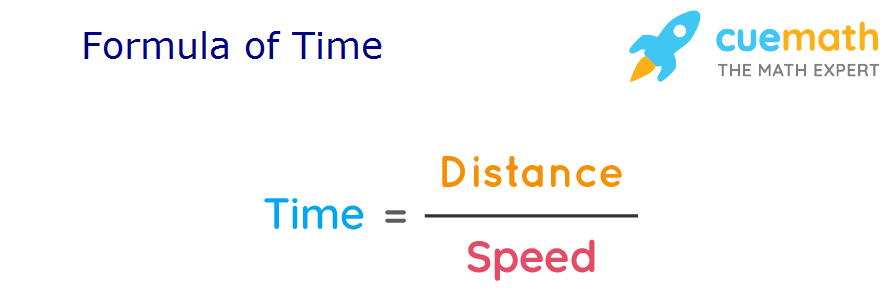# Formula of Time

Go back to  'Math Formulas'

Time can be defined as the distance an object covers at unit speed. Time refers to the progression of events. This progression is in such a manner that it goes from the past to the present and finally into the future.

The formula of time helps in calculating the time taken by anybody to travel a certain distance at a given speed. The SI unit of time is s.

Let us study the formula of time using some solved examples.

## What is the Formula of Time?

The Formula of Time of a given body can be expressed as,

Time = Distance/Speed## Solved Examples Using Formula of Time.

### A train covered a distance of 120 km at a speed of 60km/hr. What is the time taken by the train?

Solution:

To find: Time taken by the train.

Given:

Distance covered by a train = 120km

Speed of a train = 60 km/hr

Using Formula of Time,

Time = Distance/Speed

= 120/60

= 2 hr

Answer: The time taken by the train to cover 120 km at the speed of 60km/hr is 2 hours.

### A cyclist covers 20 km at the speed of 5km/hr. What is the time taken by the cyclist?

Solution:

To find: Time taken by the cyclist.

Distance covered by a cyclist =  20 km

Speed of the cyclist = 5km/hr.

Using Formula of Time,

Time = Distance/Speed

= 20/5

= 4 hr